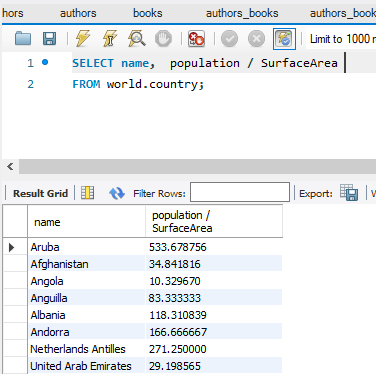1.4

# Arithmetic Operators

### Arithmetic Operators

• Arithmetic operators can be used in the SELECT, WHERE, and ORDER BY clauses.
• Operators are evaluated in the same way as arithmetic in other contexts.

Table 4. Operators and precedence order

 Operator Name Order of Precedence * Multiplication 1 / Division 1 DIV Integer Division 1 % (MOD) Modulo (remainder) 1 + Addition 2 - Subtraction 2

Code Example:

USE world;SELECT name, population / SurfaceAreaAS "People per square mile"FROM country;

Results: